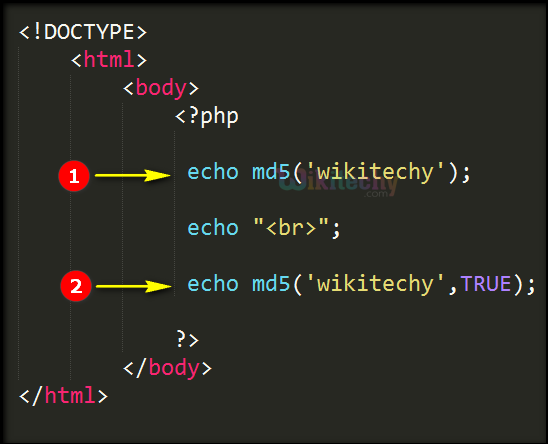# php tutorial - PHP md5 Function - php programming - learn php - php code - php script

• The md5 (message-digest algorithm) is used for calculating the hash of the string value.
• Md5 takes the input message of arbitrary length and produces as output with 128-bit "fingerprint" or "message digest" of the input.
• The MD5 algorithm is intended for digital signature applications, where a large file must be "compressed" in a secure manner before being encrypted with a private (secret) key under a public-key cryptosystem such as RSA (Rivest, Shamir, and Adelman) .

## Syntax Explanation:

• string Source - defines the input string.
• raw_output – The raw output will work like a Boolean function that is : If TRUE it sets raw 16-character binary format. If FALSE (default) it sets raw 32-character hex number format.

## Advantage of Using md5 function :

1. Utilizes a fast computation algorithm
2. Provides collision resistance
4. Provides a one-way hash

## Disadvantage of Using md5 function :

1. Has known security flaws and vulnerabilities
2. Is less secure than the SHA-1 algorithm

## php program Code Explanation :1. In this echo statement we call the “md5” string function that will be used for calculating the hash function value for the term “wikitechy” .
2. In this echo statement we call the “md5” string function that will be used for calculating the hash function value of the term wikitechy and here we use the Boolean function set as true so the raw output of the encrypted text is displayed only the 16 characters in your browser.

## php coding Sample Output :1. Output “wikitechy” is encrypted using md5 algorithm which will encrypt by the raw 32-character hex number format.
2. Output “wikitechy” is encrypted using md5 algorithm whose argument value set is true which will encrypt by the raw 16-character binary format.